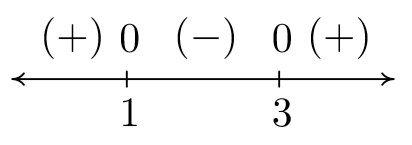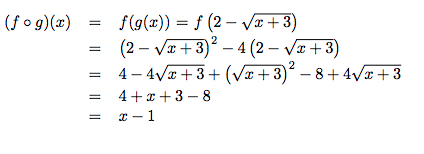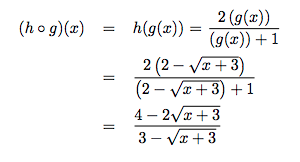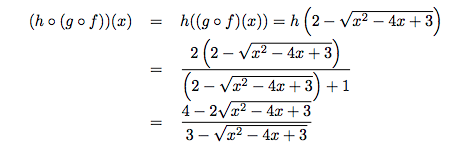$$\newcommand{\id}{\mathrm{id}}$$ $$\newcommand{\Span}{\mathrm{span}}$$ $$\newcommand{\kernel}{\mathrm{null}\,}$$ $$\newcommand{\range}{\mathrm{range}\,}$$ $$\newcommand{\RealPart}{\mathrm{Re}}$$ $$\newcommand{\ImaginaryPart}{\mathrm{Im}}$$ $$\newcommand{\Argument}{\mathrm{Arg}}$$ $$\newcommand{\norm}{\| #1 \|}$$ $$\newcommand{\inner}{\langle #1, #2 \rangle}$$ $$\newcommand{\Span}{\mathrm{span}}$$

# 5.1: Function Composition

$$\newcommand{\vecs}{\overset { \rightharpoonup} {\mathbf{#1}} }$$

$$\newcommand{\vecd}{\overset{-\!-\!\rightharpoonup}{\vphantom{a}\smash {#1}}}$$

Before we embark upon any further adventures with functions, we need to take some time to gather our thoughts and gain some perspective. Chapter 1 first introduced us to functions in Section 1.3. At that time, functions were specific kinds of relations - sets of points in the plane which passed the Vertical Line Test, Theorem 1.1. In Section 1.4, we developed the idea that functions are processes - rules which match inputs to outputs - and this gave rise to the concepts of domain and range. We spoke about how functions could be combined in Section 1.5 using the four basic arithmetic operations, took a more detailed look at their graphs in Section 1.6} and studied how their graphs behaved under certain classes of transformations in Section 1.7. In Chapter 2 we took a closer look at three families of functions: linear functions (Section 2.1), absolute value functions\footnote{These were introduced, as you may recall, as piecewise-defined linear functions.} (Section 2.2), and quadratic functions (Section 2.3). Linear and quadratic functions were special cases of polynomial functions, which we studied in generality in Chapter 3. Chapter 3 culminated with the Real Factorization Theorem, Theorem 3.16, which says that all polynomial functions with real coefficients can be thought of as products of linear and quadratic functions. Our next step was to enlarge our field \footnote{This is a really bad math pun.} of study to rational functions in Chapter 4. Being quotients of polynomials, we can ultimately view this family of functions as being built up of linear and quadratic functions as well. So in some sense, Chapters 2, 3, and 4 can be thought of as an exhaustive study of linear and quadratic \footnote{If we broaden our concept of functions to allow for complex valued , tells us every function we have studied thus far is a combination of linear functions.} functions and their arithmetic combinations as described in Section 1.5. We now wish to study other algebraic functions, such as $$f(x) = \sqrt{x}$$ and $$g(x) = x^{2/3}$$, and the purpose of the first two sections of this chapter is to see how these kinds of functions arise from polynomial and rational functions. To that end, we first study a new way to combine functions as defined below.

Definition 5.1: Compose Functions

Suppose $$f$$ and $$g$$ are two functions. The composite of $$g$$ with $$f$$, denoted $$g \circ f$$, is defined by the formula $$(g \circ f) (x) = g(f(x))$$, provided $$x$$ is an element of the domain of $$f$$ and $$f(x)$$ is an element of the domain of $$g$$.

The quantity $$g \circ f$$ is also read '$$g$$ composed with $$f$$' or, more simply '$$g$$ of $$f$$.' At its most basic level, Definition 5.1 tells us to obtain the formula for $$\left(g \circ f\right)(x)$$, we replace every occurrence of $$x$$ in the formula for $$g(x)$$ with the formula we have for $$f(x)$$. If we take a step back and look at this from a procedural, 'inputs and outputs' perspective, Defintion 5.1 tells us the output from $$g \circ f$$ is found by taking the output from $$f$$, $$f(x)$$, and then making that the input to $$g$$. The result, $$g(f(x))$$, is the output from $$g \circ f$$. From this perspective, we see $$g \circ f$$ as a two step process taking an input $$x$$ and first applying the procedure $$f$$ then applying the procedure $$g$$. Abstractly, we haveIn the expression $$g(f(x))$$, the function $$f$$ is often called the 'inside' function while $$g$$ is often called the 'outside' function. There are two ways to go about evaluating composite functions - 'inside out' and 'outside in' - depending on which function we replace with its formula first. Both ways are demonstrated in the following example.

Example $$\PageIndex{1}$$:

Let $$f(x) = x^2-4x$$, $$g(x) = 2-\sqrt{x+3}$$, and $$h(x) = \dfrac{2x}{x+1}$$.

In numbers 1 - 3, find the indicated function value.

1. $$(g \circ f)(1)$$
2. $$(f \circ g)(1)$$
3. $$(g \circ g)(6)$$

In numbers 4 - 10, find and simplify the indicated composite functions. State the domain of each.

1. $$(g \circ f)(x)$$
2. $$(f \circ g)(x)$$
3. $$(g \circ h)(x)$$
4. $$(h \circ g)(x)$$
5. $$(h \circ h)(x)$$
6. $$(h \circ (g \circ f))(x)$$
7. $$((h \circ g) \circ f)(x)$$

$$\textbf{Solution}$$

1. Using Definition 5.1, $$(g \circ f)(1) = g(f(1))$$. We find $$f(1) = -3$$, so

$(g \circ f)(1) = g(f(1)) = g(-3) = 2$

2. As before, we use Definition 5.1 to write $$(f \circ g)(1) = f(g(1))$$. We find $$g(1) = 0$$, so

$(f \circ g)(1) = f(g(1)) = f(0) = 0$

3. Once more, Definition 5.1 tells us $$(g \circ g)(6) = g(g(6))$$. That is, we evaluate $$g$$ at $$6$$, then plug that result back into $$g$$. Since $$g(6) = -1$$,

$(g \circ g)(6) = g(g(6)) = g(-1) = 2-\sqrt{2}$

4. By definition, $$(g \circ f)(x) = g(f(x))$$. We now illustrate $$\textit{two}$$ ways to approach this problem.

• $$\textit{inside out}$$: We insert the expression $$f(x)$$ into $$g$$ first to get

$(g \circ f)(x) = g(f(x)) = g\left(x^2-4x\right) = 2 - \sqrt{\left(x^2-4x\right)+3} = 2 - \sqrt{x^2-4x+3}$

Hence, $$(g \circ f)(x) = 2 - \sqrt{x^2-4x+3}$$.

• $$\textit{outside in}$$: We use the formula for $$g$$ first to get

$(g \circ f)(x) = g(f(x)) = 2 - \sqrt{f(x)+3} = 2 - \sqrt{\left(x^2-4x\right)+3} = 2 - \sqrt{x^2-4x+3}$

We get the same answer as before, $$(g \circ f)(x) = 2 - \sqrt{x^2-4x+3}$$.

To find the domain of $$g \circ f$$, we need to find the elements in the domain of $$f$$ whose outputs $$f(x)$$ are in the domain of $$g$$. We accomplish this by following the rule set forth in Section 1.4, that is, we find the domain $$\textit{before}$$ we simplify. To that end, we examine $$(g \circ f)(x) = 2 - \sqrt{\left(x^2-4x\right)+3}$$. To keep the square root happy, we solve the inequality $$x^2-4x+3 \geq 0$$ by creating a sign diagram. If we let $$r(x) = x^2-4x+3$$, we find the zeros of $$r$$ to be $$x = 1$$ and $$x = 3$$. We obtainOur solution to $$x^2-4x+3 \geq 0$$, and hence the domain of $$g \circ f$$, is $$(-\infty, 1] \cup [3,\infty)$$.

5. To find $$(f \circ g)(x)$$, we find $$f(g(x))$$.

• $$\textit{inside out}$$: We insert the expression $$g(x)$$ into $$f$$ first to get• $$\textin{outside in}$$ : We use the formula for $$f(x)$$ first to getThus we get $$(f \circ g)(x) = x-1$$. To find the domain of $$(f \circ g)$$, we look to the step before we did any simplification and find $$(f \circ g)(x) = \left(2-\sqrt{x+3}\right)^2 - 4\left(2-\sqrt{x+3}\right)$$. To keep the square root happy, we set $$x+3 \geq 0$$ and find our domain to be $$[-3, \infty)$$.

6. To find $$(g \circ h)(x)$$, we compute $$g(h(x))$$.

• $$\textit{inside out}$$: We insert the expression $$h(x)$$ into $$g$$ first to get• $$\textit{outside in}$$: We use the formula for $$g(x)$$ first to getTo find the domain of $$(g \circ h)$$, we look to the step before we began to simplify:

$(g \circ h)(x) = 2 - \sqrt{\left(\frac{2x}{x+1}\right)+3}$

To avoid division by zero, we need $$x \neq -1$$. To keep the radical happy, we need to solve

$\frac{2x}{x+1} +3 = \frac{5x+3}{x+1}\geq 0$

Defining $$r(x) = \frac{5x+3}{x+1}$$, we see $$r$$ is undefined at $$x=-1$$ and $$r(x) = 0$$ at $$x = -\frac{3}{5}$$. We get

Our domain is $$(-\infty, -1) \cup \left[-\frac{3}{5}, \infty\right)$$.

7. We find $$(h \circ g)(x)$$ by finding $$h(g(x))$$.

• $$\textit{inside out}$$: We insert the expression $$g(x)$$ into $$h$$ first to get• $$\textit{outside in}$$: We use the formula for $$h(x)$$ first to getTo find the domain of $$h \circ g$$, we look to the step before any simplification:

$(h \circ g)(x) = \frac{2 \left(2-\sqrt{x+3} \right)}{\left(2-\sqrt{x+3}\right)+1}$

To keep the square root happy, we require $$x+3 \geq 0$$ or $$x \geq -3$$. Setting the denominator equal to zero gives $$\left(2-\sqrt{x+3}\right)+1=0$$ or $$\sqrt{x+3} = 3$$. Squaring both sides gives us $$x+3=9$$, or $$x=6$$. Since $$x=6$$ checks in the original equation, $$\left(2-\sqrt{x+3}\right)+1=0$$, we know $$x=6$$ is the only zero of the denominator. Hence, the domain of $$h \circ g$$ is $$[-3,6) \cup (6, \infty)$$.

8. To find $$(h \circ h)(x)$$, we substitute the function $$h$$ into itself, $$h(h(x))$$.

• $$\textit{inside out}$$: We insert the expression $$h(x)$$ into $$h$$ to get• $$\textit{outside in}$$: This approach yieldsTo find the domain of $$h \circ h$$, we analyze

$(h \circ h)(x) = \dfrac{2\left(\dfrac{2x}{x+1}\right)}{\left(\dfrac{2x}{x+1}\right)+1}$

To keep the denominator $$x+1$$ happy, we need $$x \neq -1$$. Setting the denominator

$\frac{2x}{x+1}+1 = 0$ gives $$x = -\frac{1}{3}$$.

Our domain is $$(-\infty, -1) \cup \left(-1, -\frac{1}{3}\right) \cup \left(-\frac{1}{3}, \infty\right)$$.

9. The expression $$(h \circ (g \circ f))(x)$$ indicates that we first find the composite, $$g \circ f$$ and compose the function $$h$$ with the result. We know from number 1 that $$(g \circ f)(x) = 2 - \sqrt{x^2-4x+3}$$. We now proceed as usual.

• $$\textit{inside out}$$: We insert the expression $$(g \circ f)(x)$$ into $$h$$ first to get• $$\textit{outside in}$$: We use the formula for $$h(x)$$ first to getTo find the domain of $$(h \circ (g \circ f))$$, we look at the step before we began to simplify,

$(h \circ (g \circ f))(x) = \frac{2 \left(2 - \sqrt{x^2-4x+3}\right)}{\left(2 - \sqrt{x^2-4x+3}\right)+1}$

For the square root, we need $$x^2-4x+3 \geq 0$$, which we determined in number 1 to be $$(-\infty, 1] \cup [3,\infty)$$. Next, we set the denominator to zero and solve: $$\left(2 - \sqrt{x^2-4x+3}\right)+1 = 0$$. We get $$\sqrt{x^2-4x+3} = 3$$, and, after squaring both sides, we have $$x^2-4x+3 = 9$$. To solve $$x^2-4x-6 = 0$$, we use the quadratic formula and get $$x = 2 \pm \sqrt{10}$$. The reader is encouraged to check that both of these numbers satisfy the original equation, $$\left(2 - \sqrt{x^2-4x+3}\right)+1 = 0$$. Hence we must exclude these numbers from the domain of $$h \circ (g \circ f)$$. Our final domain for $$h \circ (f \circ g)$$ is $$(-\infty, 2 -\sqrt{10}) \cup (2 - \sqrt{10}, 1] \cup \left[3, 2 + \sqrt{10}\right) \cup \left(2+\sqrt{10}, \infty\right)$$.

10. The expression $$((h \circ g) \circ f)(x)$$ indicates that we first find the composite $$h \circ g$$ and then compose that with $$f$$. From number 4, we have

$(h \circ g)(x) = \frac{4-2\sqrt{x+3}}{3-\sqrt{x+3}}$

We now proceed as before.

• $$\textit{inside out}$$: We insert the expression $$f(x)$$ into $$h \circ g$$ first to get

$\begin{array}{rclr} ((h \circ g) \circ f)(x) & = & (h \circ g)(f(x)) = (h \circ g)\left(x^2-4x\right) & \\ & = & \dfrac{4-2\sqrt{\left(x^2-4x\right)+3}}{3-\sqrt{\left(x^2-4x\right)+3}} & \\ & = & \dfrac{4 - 2\sqrt{x^2-4x+3}}{3 - \sqrt{x^2-4x+3}} & \\ \end{array}$

• $$\textit{outside in}$$: We use the formula for $$(h \circ g)(x)$$ first to getWe note that the formula for $$((h \circ g) \circ f)(x)$$ before simplification is identical to that of $$(h \circ (g \circ f))(x)$$ before we simplified it. Hence, the two functions have the same domain, $$h \circ (f \circ g)$$ is $$(-\infty, 2 -\sqrt{10}) \cup (2 - \sqrt{10}, 1] \cup \left[3, 2 + \sqrt{10}\right) \cup \left(2+\sqrt{10}, \infty\right)$$. $$\Box$$

It should be clear from Example 5.1.1that, in general, when you compose two functions, such as $$f$$ and $$g$$ above, the order matters.\footnote{This shows us function composition isn't $$\textbf{commutative}$$. \index{commutative property ! function composition does not have} An example of an operation we perform on two functions which is commutative is function addition, which we defined in Section 1.5. In other words, the functions $$f+g$$ and $$g+f$$ are always equal. Which of the remaining operations on functions we have discussed are commutative?} We found that the functions $$f \circ g$$ and $$g \circ f$$ were different as were $$g \circ h$$ and $$h \circ g$$. Thinking of functions as processes, this isn't all that surprising. If we think of one process as putting on our socks, and the other as putting on our shoes, the order in which we do these two tasks does matter. \footnote{A more mathematical example in which the order of two processes matters can be found in Section1.7. In fact, all of the transformations in that section can be viewed in terms of composing functions with linear functions.} Also note the importance of finding the domain of the composite function $$\textit{before}$$ simplifying. For instance, the domain of $$f \circ g$$ is much different than its simplified formula would indicate. Composing a function with itself, as in the case of finding $$(g\circ g)(6)$$ and $$(h \circ h)(x)$$, may seem odd. Looking at this from a procedural perspective, however, this merely indicates performing a task $$h$$ and then doing it again - like setting the washing machine to do a 'double rinse'. Composing a function with itself is called 'iterating' the function, and we could easily spend an entire course on just that. The last two problems in Example 5.1.1 serve to demonstrate the \index{associative property ! for function composition} $$\textbf{associative}$$ property of functions. That is, when composing three (or more) functions, as long as we keep the order the same, it doesn't matter which two functions we compose first. This property as well as another important property are listed in the theorem below.

Theorem 5.1: Properties of Function Composition

Suppose $$f$$, $$g$$, and $$h$$ are functions.

• $$h \circ (g \circ f) = (h \circ g) \circ f$$, provided the composite functions are defined.
• If $$I$$ is defined as $$I(x) = x$$ for all real numbers $$x$$, then $$I \circ f = f \circ I =f$$.

By repeated applications of Definition 5.1, we find $$(h \circ (g \circ f))(x) = h((g \circ f)(x)) = h(g(f(x)))$$. Similarly, $$((h \circ g) \circ f)(x) = (h \circ g)(f(x)) = h(g(f(x)))$$. This establishes that the formulas for the two functions are the same. We leave it to the reader to think about why the domains of these two functions are identical, too. These two facts establish the equality $$h \circ (g \circ f) = (h \circ g) \circ f$$. A consequence of the associativity of function composition is that there is no need for parentheses when we write $$h \circ g \circ f$$. The second property can also be verified using Definition 5.1. Recall that the function $$I(x) = x$$ is called the \index{identity ! function} \textit{identity function} and was introduced in Exercise \ref{identityexercise} in Section 2.1. If we compose the function $$I$$ with a function $$f$$, then we have $$(I \circ f)(x) = I(f(x)) = f(x)$$, and a similar computation shows $$(f\circ I)(x) = f(x)$$. This establishes that we have an identity for function composition much in the same way the real number $$1$$ is an identity for real number multiplication. That is, just as for any real number $$x$$, $$1 \cdot x = x \cdot 1 = x$$ , we have for any function $$f$$, $$I \circ f = f \circ I =f$$. We shall see the concept of an identity take on great significance in the next section. Out in the wild, function composition is often used to relate two quantities which may not be directly related, but have a variable in common, as illustrated in our next example.

Example $$\PageIndex{2}$$

The surface area $$S$$ of a sphere is a function of its radius $$r$$ and is given by the formula $$S(r) = 4 \pi r^2$$. Suppose the sphere is being inflated so that the radius of the sphere is increasing according to the formula $$r(t) = 3t^2$$, where $$t$$ is measured in seconds, $$t \geq 0$$, and $$r$$ is measured in inches. Find and interpret $$(S \circ r)(t)$$.

$$\textbf{Solution}$$

If we look at the functions $$S(r)$$ and $$r(t)$$ individually, we see the former gives the surface area of a sphere of a given radius while the latter gives the radius at a given time. So, given a specific time, $$t$$, we could find the radius at that time, $$r(t)$$ and feed that into $$S(r)$$ to find the surface area at that time. From this we see that the surface area $$S$$ is ultimately a function of time $$t$$ and we find $$(S \circ r)(t) = S(r(t)) = 4 \pi (r(t))^2 = 4 \pi \left(3t^2\right)^2 = 36 \pi t^{4}$$. This formula allows us to compute the surface area directly given the time without going through the 'middle man' $$r$$. $$\Box$$

A useful skill in Calculus is to be able to take a complicated function and break it down into a composition of easier functions which our last example illustrates.

Example $$\PageIndex{3}$$

Write each of the following functions as a composition of two or more (non-identity) functions. Check your answer by performing the function composition.

1. $$F(x) = |3x-1|$$
2. $$G(x) = \dfrac{2}{x^2+1}$$
3. $$H(x) = \dfrac{\sqrt{x}+1}{\sqrt{x}-1}$$

$$\textbf{Solution}$$

There are many approaches to this kind of problem, and we showcase a different methodology in each of the solutions below.

1. Our goal is to express the function $$F$$ as $$F = g \circ f$$ for functions $$g$$ and $$f$$. From Definition 5.1, we know $$F(x) = g(f(x))$$, and we can think of $$f(x)$$ as being the 'inside' function and $$g$$ as being the 'outside' function. Looking at $$F(x) = |3x-1|$$ from an 'inside versus outside' perspective, we can think of $$3x-1$$ being inside the absolute value symbols. Taking this cue, we define $$f(x) = 3x-1$$. At this point, we have $$F(x) = |f(x)|$$. What is the outside function? The function which takes the absolute value of its input, $$g(x) = |x|$$. Sure enough, $$(g \circ f)(x) = g(f(x)) = |f(x)| = |3x-1| = F(x)$$, so we are done.
2. We attack deconstructing $$G$$ from an operational approach. Given an input $$x$$, the first step is to square $$x$$, then add $$1$$, then divide the result into $$2$$. We will assign each of these steps a function so as to write $$G$$ as a composite of three functions: $$f$$, $$g$$ and $$h$$. Our first function, $$f$$, is the function that squares its input, $$f(x) = x^2$$. The next function is the function that adds $$1$$ to its input, $$g(x) = x+1$$. Our last function takes its input and divides it into $$2$$, $$h(x) = \frac{2}{x}$$. The claim is that $$G = h \circ g \circ f$$. We find $(h \circ g \circ f)(x) = h(g(f(x))) = h(g\left(x^2\right)) = h\left(x^2+1\right)= \frac{2}{x^2+1} = G(x),$ so we are done.
3. If we look $$H(x) = \frac{\sqrt{x}+1}{\sqrt{x}-1}$$ with an eye towards building a complicated function from simpler functions, we see the expression $$\sqrt{x}$$ is a simple piece of the larger function. If we define $$f(x) = \sqrt{x}$$, we have $$H(x) = \frac{f(x)+1}{f(x)-1}$$. If we want to decompose $$H = g \circ f$$, then we can glean the formula for $$g(x)$$ by looking at what is being done to $$f(x)$$. We take $$g(x) = \frac{x+1}{x-1}$$, so $(g \circ f)(x) = g(f(x)) = \frac{f(x)+1}{f(x)-1} = \frac{\sqrt{x}+1}{\sqrt{x}-1} = H(x),$ as required. $$\Box$$

## Contributors

• Carl Stitz, Ph.D. (Lakeland Community College) and Jeff Zeager, Ph.D. (Lorain County Community College)